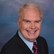# Math Solutions: Volume CalculationsBy Daniel Theobald, "Wastewater Dan"

Water Online’s “Math Solutions” series, presented by wastewater consultant and trainer Dan Theobald (“Wastewater Dan”), provides an understanding of the sometimes difficult calculations involved in achieving wastewater operator certification.

Volume calculations may have different requirements. Results may be expressed as cubic feet, increment of millions, or even incorporating a time element in calculation solutions.

Depending on the calculation used with volume, calculations certain pipes or tanks (dictated by size/shape) may require explicit formula use.

Some common volume calculations are accessible below:

Calculate Volume

Cubic feet in a treatment tank:

Calculate volume in million gallons (MG):

Calculate cubic feet per second:

Calculate pipe volume in gallons:

Calculate gallons of sludge in a conical bottom circular tank:

This is the flow rate conversion calculations presentation in my series of “Math Solutions” (access the full playlist here). If you have specific wastewater math queries, please submit a question.